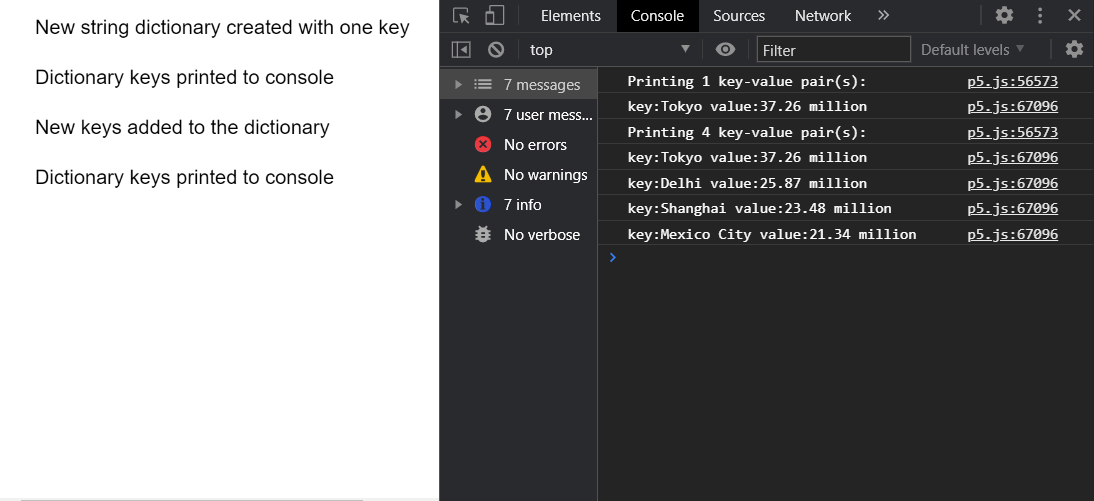Related Articles
p5.js TypedDict print() Method
• Last Updated : 27 Nov, 2020

The print() method of p5.TypedDict in p5.js is used to print out all the key-value pairs currently present in the dictionary to the console. A key-value pair is a set of two values that are mapped to each other. These values can be accessed by querying this dictionary using the key portion of the pair. A typed dictionary can store multiple key-value pairs that can be accessed using the methods of the dictionary.

Syntax:

```print()
```

Parameters: This method does not accept any parameters.

The example below illustrates the print() method in p5.js:

Example:

## Javascript

 `function` `setup() { ` `  ``createCanvas(550, 300); ` `  ``textSize(16); ` ` `  `  ``let stringDict =  ` `      ``createStringDict(``"Tokyo"``, ``"37.26 million"``); ` `  ``text(``"New string dictionary created "` `+ ` `       ``"with one key"``, 20, 20); ` ` `  `  ``// Getting the current size of the dictionary ` `  ``let currSize = stringDict.size(); ` `  ``print(``"Printing "` `+ currSize + ` `        ``" key-value pair(s):"``); ` ` `  `  ``// Printing all the values to console ` `  ``stringDict.print(); ` `  ``text(``"Dictionary keys printed to console"``, ` `       ``20, 60); ` ` `  `  ``let tmpObj = { ` `    ``"Delhi"``: ``"25.87 million"``, ` `    ``"Shanghai"``: ``"23.48 million"``, ` `    ``"Mexico City"``: ``"21.34 million"` `  ``}; ` ` `  `  ``// Add the given key to the dictionary ` `  ``// specifying the key and value as an object ` `  ``stringDict.create(tmpObj); ` `  ``text(``"New keys added to the dictionary"``, ` `       ``20, 100); ` ` `  `  ``// Getting the current size of the dictionary ` `  ``currSize = stringDict.size(); ` `  ``print(``"Printing "` `+ currSize + ` `        ``" key-value pair(s):"``); ` ` `  `  ``// Printing all the values to console ` `  ``stringDict.print(); ` `  ``text(``"Dictionary keys printed to console"``, ` `       ``20, 140); ` `} `

Output:Online editor: https://editor.p5js.org/
Environment Setup: https://www.geeksforgeeks.org/p5-js-soundfile-object-installation-and-methods/
Reference: https://p5js.org/reference/#/p5.TypedDict/printMy Personal Notes arrow_drop_up
Recommended Articles
Page :